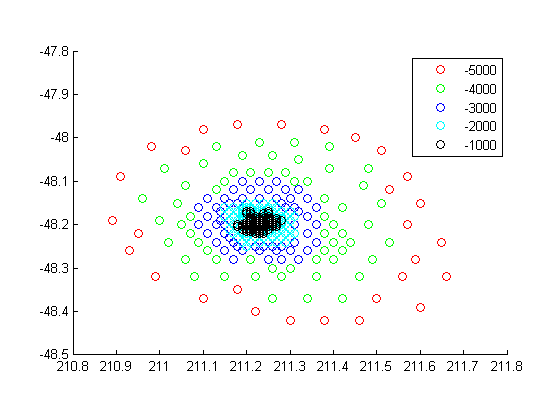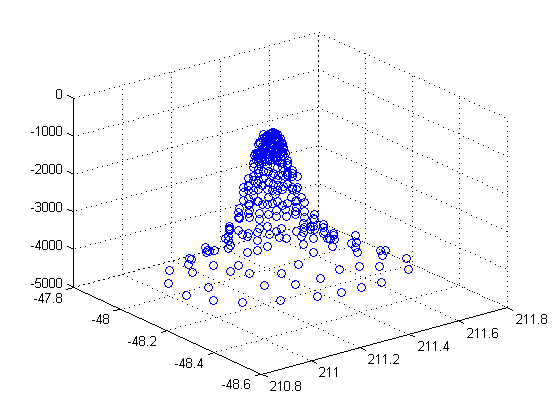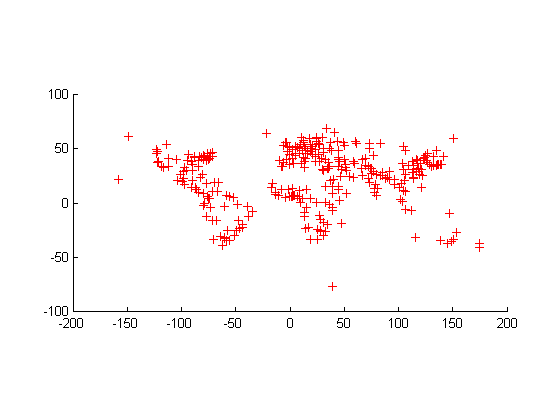mappoint

Planar point vector

Description

A mappoint vector is a container object that holds planar point coordinates and attributes. The points are coupled, such that the size of the x- and y-coordinate arrays are always equal and match the size of any dynamically added attribute arrays. Each entry of a coordinate pair and associated attributes, if any, represents a discrete element in the mappoint vector.

To create a planar point shape for use with a geospatial table, create a mappointshape object instead.

Creation

Description

example

p = mappoint() constructs an empty mappoint vector, p, with these default property settings:

p =

0x1 mappoint vector with properties:

Collection properties:
Geometry: 'point'
Feature properties:
X: []
Y: []

example

p = mappoint(x,y) constructs a new mappoint vector and assigns the X and Y properties to the numeric array inputs, x and y.

example

p = mappoint(x,y,Name,Value) constructs a mappoint vector, then adds dynamic properties to the mappoint vector using Name, Value argument pairs. You can specify several name-value pair arguments in any order as Name1,Value1,...,NameN,ValueN.

example

p = mappoint(structArray) constructs a new mappoint vector, assigning the fields of the structure array, structArray, as dynamic properties. Field values in structArray that are not numeric values, string scalar, string array, character vectors, or cell arrays of numeric values or character vectors are ignored.

example

p = mappoint(x,y,structArray) constructs a new mappoint vector, sets the X and Y properties equal to the numeric arrays x and y, and sets dynamic properties from the field values of structArray.

Properties

expand all

Each element in a mappoint vector is considered a feature. For more about the property types in mappoint, see Collection Properties and Feature Properties.

Dynamic properties are new features that are added to a mappoint vector and that apply to each individual feature in the mappoint vector. You can attach dynamic Feature properties to a mappoint object during construction with a Name,Value pair or after construction using dot (.) notation. This is similar to adding dynamic fields to a structure. For an example of adding Feature properties dynamically, see Construct a Mappoint Vector for Multiple Features and Examine Autosizing.

Type of geometry, specified as 'point'. For mappoint, Geometry is always 'point'.

Data Types: char | string

Information for the entire set of mappoint vector elements, specified as a scalar structure. You can add any data type to the structure.

• If Metadata is provided as a dynamic property Name in the constructor, and the corresponding Value is a scalar structure, then Value is copied to the Metadata property. Otherwise, an error is issued.

• If a Metadata field is provided by structArray, and both Metadata and structArray are scalar structures, then the Metadata field value is copied to the Metadata property value. If structArray is a scalar but the Metadata field is not a structure, then an error is issued. If structArray is not scalar, then the Metadata field is ignored.

Data Types: struct

Planar x-coordinate, specified as a numeric row or column vector.

Data Types: double | single

Planar y-coordinates, specified as a numeric row or column vector.

Data Types: double | single

Object Functions

 append Append features to geographic or planar vector cat Concatenate geographic or planar vector disp Display geographic or planar vector fieldnames Return dynamic property names of geographic or planar vector isempty Determine if geographic or planar vector is empty isfield Determine if dynamic property exists in geographic or planar vector isprop Determine if property exists in geographic or planar vector length Return number of elements in geographic or planar vector properties Return property names of geographic or planar vector rmfield Remove dynamic property from geographic or planar vector rmprop Remove property from geographic or planar vector size Return size of geographic or planar vector struct Convert geographic or planar vector to scalar structure vertcat Vertically concatenate geographic or planar vectors

Examples

collapse all

Dynamically set the X and Y property values, and dynamically add Vertex property Z.

p = mappoint();
p.X = 1:3;
p.Y = 1:3;
p.Z = [10 10 10]
p =

3x1 mappoint vector with properties:

Collection properties:
Geometry: 'point'
Feature properties:
X: [1 2 3]
Y: [1 2 3]
Z: [10 10 10]

Define x and y coordinates. Use them to create a mappoint.

x = [40 50 60];
y = [10, 11, 12];
p = mappoint(x, y)
p =

3x1 mappoint vector with properties:

Collection properties:
Geometry: 'point'
Feature properties:
X: [40 50 60]
Y: [10 11 12]
x = 41:43;
y = 1:3;
temperature = 61:63;
p = mappoint(x, y, 'Temperature', temperature)
p =

3x1 mappoint vector with properties:

Collection properties:
Geometry: 'point'
Feature properties:
X: [41 42 43]
Y: [1 2 3]
Temperature: [61 62 63]

Create a structure array and then create a mappoint vector, specifying the array as input.

p = mappoint(structArray)
structArray =

13x1 struct array with fields:
Geometry
X
Y
NAME
FEATURE
COORD

p =

13x1 mappoint vector with properties:

Collection properties:
Geometry: 'point'
Feature properties:
X: [1x13 double]
Y: [1x13 double]
NAME: {1x13 cell}
FEATURE: {1x13 cell}
COORD: {1x13 cell
x = [structArray.X];
y = [structArray.Y];
p = mappoint(x, y, A)
p =

13x1 mappoint vector with properties:

Collection properties:
Geometry: 'point'
Feature properties:
X: [1x13 double]
Y: [1x13 double]
NAME: {1x13 cell}
FEATURE: {1x13 cell}
COORD: {1x13 cell}

This example shows how to add a single feature to an empty mappoint vector after construction.

x = 1;
y = 1;
p = mappoint(x, y)
p =

1x1 mappoint vector with properties:

Collection properties:
Geometry: 'point'
Feature properties:
X: 1
Y: 1

Add a dynamic Feature property with a character vector value.

p.FeatureName = 'My Feature'
p =

1x1 mappoint vector with properties:

Collection properties:
Geometry: 'point'
Feature properties:
X: 1
Y: 1
FeatureName: 'My Feature'

This example show how mappoint vectors autoresize all properties lengths to ensure they are equal in size when a new dynamic property is added or an existing property is appended or shortened.

Create a mappoint vector.

x = [1 2];
y = [10 10];
p = mappoint(x,y)
p =

2x1 mappoint vector with properties:

Collection properties:
Geometry: 'point'
Feature properties:
X: [1 2]
Y: [10 10]

p.FeatureName = {'Feature 1','Feature 2'}
p =

2x1 mappoint vector with properties:

Collection properties:
Geometry: 'point'
Feature properties:
X: [1 2]
Y: [10 10]
FeatureName: {'Feature 1' 'Feature 2'}

Add a numeric dynamic Feature property.

p.ID = [1 2]
p =

2x1 mappoint vector with properties:

Collection properties:
Geometry: 'point'
Feature properties:
X: [1 2]
Y: [10 10]
FeatureName: {'Feature 1' 'Feature 2'}
ID: [1 2]

Add a third feature. All properties are autosized so that all vector lengths match.

p(3).X = 3
p(3).Y = 10
p =

3x1 mappoint vector with properties:

Collection properties:
Geometry: 'point'
Feature properties:
X: [1 2 3]
Y: [10 10 10]
FeatureName: {'Feature 1' 'Feature 2' ''}
ID: [1 2 0]

Set the values for the ID feature dynamic property with more values than contained in X or Y. All properties are expanded to match in size.

p.ID = 1:4
p =

4x1 mappoint vector with properties:

Collection properties:
Geometry: 'point'
Feature properties:
X: [1 2 3 0]
Y: [10 10 10 0]
FeatureName: {'Feature 1' 'Feature 2' '' ''}
ID: [1 2 3 4]

Set the values for the ID dynamic Feature property with fewer values than contained in X or Y. The ID property values expand to match the length of X and Y.

p.ID = 1:2
p =

4x1 mappoint vector with properties:

Collection properties:
Geometry: 'point'
Feature properties:
X: [1 2 3 0]
Y: [10 10 10 0]
FeatureName: {'Feature 1' 'Feature 2' '' ''}
ID: [1 2 0 0]

Set the values of either coordinate property (X or Y) with fewer values. All properties shrink in size to match the new length.

p.X = 1:2
p =

2x1 mappoint vector with properties:

Collection properties:
Geometry: 'point'
Feature properties:
X: [1 2]
Y: [10 10]
FeatureName: {'Feature 1' 'Feature 2'}
ID: [1 2]

Remove the FeatureName property by setting its value to [ ].

p.FeatureName = []
p =

2x1 mappoint vector with properties:

Collection properties:
Geometry: 'point'
Feature properties:
X: [1 2]
Y: [10 10]
ID: [1 2]

Remove all dynamic properties and set the object to empty by setting a coordinate property value to [ ].

p.X = []
Collection properties:
Geometry: 'point'
Feature properties:
X: []
Y: []

This example shows how to include multiple dynamic features during object construction.

point = mappoint([42 44],[10, 11],'Temperature',[63 65], ...
'TemperatureUnits','Fahrenheit')
point =

2x1 mappoint vector with properties:

Collection properties:
Geometry: 'point'
Feature properties:
X: [42 44]
Y: [10 11]
Temperature: [63 65]
TemperatureUnits: 'Fahrenheit'

This example shows how to construct a mappoint vector using data from a MAT-file containing oceanic depths.

Load data from the seamount MAT-file and construct a mappoint vector to hold the coordinates.

p = mappoint(seamount.x, seamount.y, 'Z', seamount.z);

Create a level list to use to bin the z values and create a list of color values for each level.

levels = [unique(floor(seamount.z/1000)) * 1000; 0];
colors = {'red','green','blue','cyan','black'};

Add a MinLevel and MaxLevel feature property to indicate the lowest and highest binned level. Add a dynamic feature property to indicate the z-coordinate. Add a dynamic Feature property to indicate a binned level value and a color value for a given level. Include metadata information from the MAT-file.

for k = 1:length(levels) - 1
index = levels(k) <= p.Z & p.Z < levels(k+1);
p(index).MinLevel = levels(k);
p(index).MaxLevel = levels(k+1) - 1;
p(index).Color = colors{k};
end

Add metadata information. Metadata is a scalar structure containing information for the entire set of properties. You can add any type of data to the structure.

ans =

Caption: [1x229 char]

Display the point data as a 2-D plot.

figure
minLevels = unique(p.MinLevel);
for k=1:length(minLevels)
index = p.MinLevel == minLevels(k);
mapshow(p(index).X, p(index).Y, ...
'MarkerEdgeColor', p(find(index,1)).Color, ...
'Marker', 'o', ...
'DisplayType', 'point')
end
legend(num2str(minLevels'))Display the point data as a 3-D scatter plot.

figure
scatter3(p.X, p.Y, p.Z)This example shows how to create a mappoint vector from a structure array, and how to add features and metadata to the mappoint vector.

p = mappoint();
p.X = [structArray.X];
p.Y = [structArray.Y];
p.Name = {structArray.NAME}
p =

13x1 mappoint vector with properties:

Collection properties:
Geometry: 'point'
Feature properties:
X: [1x13 double]
Y: [1x13 double]
Name: {1x13 cell}

Construct a mappoint vector from a structure array using the constructor syntax.

filename = 'boston_placenames.shp';
p = mappoint(structArray)
p =

13x1 mappoint vector with properties:

Collection properties:
Geometry: 'point'
Feature properties:
X: [1x13 double]
Y: [1x13 double]
NAME: {1x13 cell}
FEATURE: {1x13 cell}
COORD: {1x13 cell}

Add a Filename field to the Metadata structure. Display the first five points and the Metadata structure.

p(1:5)
ans =

5x1 mappoint vector with properties:

Collection properties:
Geometry: 'point'
Feature properties:
X: [2.3403e+05 2.3357e+05 2.3574e+05 2.3627e+05 2.3574e+05]
Y: [900038 9.0019e+05 9.0113e+05 9.0097e+05 9.0036e+05]
NAME: {1x5 cell}
FEATURE: {'PPL-SUBDVSN' ' MARSH' ' HILL' ' PPL' ' PENINSULA'}
COORD: {1x5 cell}

ans =

Filename: 'boston_placenames.shp'

This example show how to add a feature to the mappoint vector using linear indexing.

Append Paderborn, Germany to the vector of world cities.

x = 51.715254;
y = 8.75213;
p = append(p, x, y, 'Name', 'Paderborn');
p(end)
ans =

1x1 mappoint vector with properties:

Collection properties:
Geometry: 'point'
Feature properties:
X: 51.7153
Y: 8.7521

You can also add a point to the end of the mappoint vector using linear indexing. Add Arlington, Virginia to the end of the vector.

p(end+1).X = 38.880043;
p(end).Y = -77.196676;
p(end).Name = 'Arlington';
p(end-1:end)
ans =

2x1 mappoint vector with properties:

Collection properties:
Geometry: 'point'
Feature properties:
X: [51.7153 38.8800]
Y: [8.7521 -77.1967]

% Plot the points
figure
mapshow(p.X, p.Y, 'DisplayType', 'point')This example show how features can be sorted by using the indexing behavior of the mappoint class.

Construct a mappoint vector and sort the dynamic properties.

p = p(:, sort(fieldnames(p)))
p =

162x1 mappoint vector with properties:

Collection properties:
Geometry: 'point'
Feature properties:
X: [1x162 double]
Y: [1x162 double]
Cause: {1x162 cell}
Cause_Code: [1x162 double]
Country: {1x162 cell}
Day: [1x162 double]
Desc_Deaths: [1x162 double]
Eq_Mag: [1x162 double]
Hour: [1x162 double]
Iida_Mag: [1x162 double]
Intensity: [1x162 double]
Location: {1x162 cell}
Max_Height: [1x162 double]
Minute: [1x162 double]
Month: [1x162 double]
Num_Deaths: [1x162 double]
Second: [1x162 double]
Val_Code: [1x162 double]
Validity: {1x162 cell}
Year: [1x162 double]

Modify the mappoint vector to contain only the dynamic properties, 'Year', 'Month', 'Day', 'Hour', 'Minute'.

p = p(:, {'Year', 'Month', 'Day', 'Hour', 'Minute'})
p =

162x1 mappoint vector with properties:

Collection properties:
Geometry: 'point'
Feature properties:
X: [1x162 double]
Y: [1x162 double]
Year: [1x162 double]
Month: [1x162 double]
Day: [1x162 double]
Hour: [1x162 double]
Minute: [1x162 double]

Display the first five elements.

p(1:5)
ans =

5x1 mappoint vector with properties:

Collection properties:
Geometry: 'point'
Feature properties:
X: [128.3000 -156 157.9500 143.8500 -155]
Y: [-3.8000 19.5000 -9.0200 42.1500 19.1000]
Year: [1950 1951 1951 1952 1952]
Month: [10 8 12 3 3]
Day: [8 21 22 4 17]
Hour: [3 10 NaN 1 3]
Minute: [23 57 NaN 22 58]

This example demonstrates that input arguments x and y can be either row or column vectors.

If you typically store x- and y-coordinate values in an n-by-2 or 2-by-m array, you can assign a mappoint object to these numeric values. If the values are stored in an n-by-2 array, then the X property values are assigned to the first column and the Y property values are assigned to the second column.

x = 1:10;
y = 21:30;
pts = [x' y'];
p = mappoint;
p(1:length(pts)) = pts
p =

10x1 mappoint vector with properties:

Collection properties:
Geometry: 'point'
Feature properties:
X: [1 2 3 4 5 6 7 8 9 10]
Y: [21 22 23 24 25 26 27 28 29 30]

If the values are stored in a 2-by-m array, then the X property values are assigned to the first row and the Y property values are assigned to the second row.

pts = [x; y];
p(1:length(pts)) = pts
p =

10x1 mappoint vector with properties:

Collection properties:
Geometry: 'point'
Feature properties:
X: [1 2 3 4 5 6 7 8 9 10]
Y: [21 22 23 24 25 26 27 28 29 30]

Observe that in both cases, X and Y are stored as row vectors.

expand all

Tips

• If X, Y, or a dynamic property is set with more values than features in the mappoint vector, then all other properties expand in size using 0 for numeric values and an empty character vector ('') for cell values.

• If a dynamic property is set with fewer values than the number of features, then this dynamic property expands to match the size of the other properties, by inserting a 0 if the value is numeric or an empty character vector (''), if the value is a cell array.

• If the X or Y property of the mappoint vector is set with fewer values than contained in the object, then all other properties shrink in size.

• If either X or Y is set to [ ], then both coordinate properties are set to [ ] and all dynamic properties are removed.

• If a dynamic property is set to [ ], then it is removed from the object.

• The mappoint vector can be indexed like any MATLAB® vector. You can access any element of the vector to obtain a specific feature. The following examples demonstrate this behavior:

Objects

Introduced in R2012a

Mapping Toolbox DocumentationGet trial now